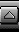Sheet 3 - Modelling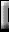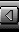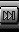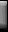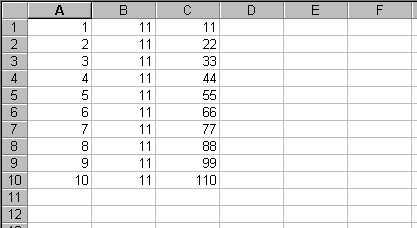You have set up the table shown above to model the eleven times table for numbers from 1 to 10.

1. What type of computer program did you use for this kind of modelling?
2. What is the reference for the cell that contains the number 2?
3. Give the formula that is required to work out the answer given in cell C1.
4. Describe how you copied the contents of C1 to produce all the answers in column C.
5. Which cell or cells would you need to change in order to model the twelve times table?
6. In a similar way, this program could be used to model the takeoff of an aeroplane and work out how much fuel is needed. Calculations would involve the mass of the craft, number of passengers, mass of the fuel, wind speed, etc. Give one reason why it is better to use a program similar to the above rather than using a simple pocket calculator.
7. A more complex program could display the answers as exact replicas of the dials you would have in front of you if you were sitting in the pilot seat. It could even simulate the view that you would see out of the window. Give one reason why it is better to use such a program for the early training of pilots.